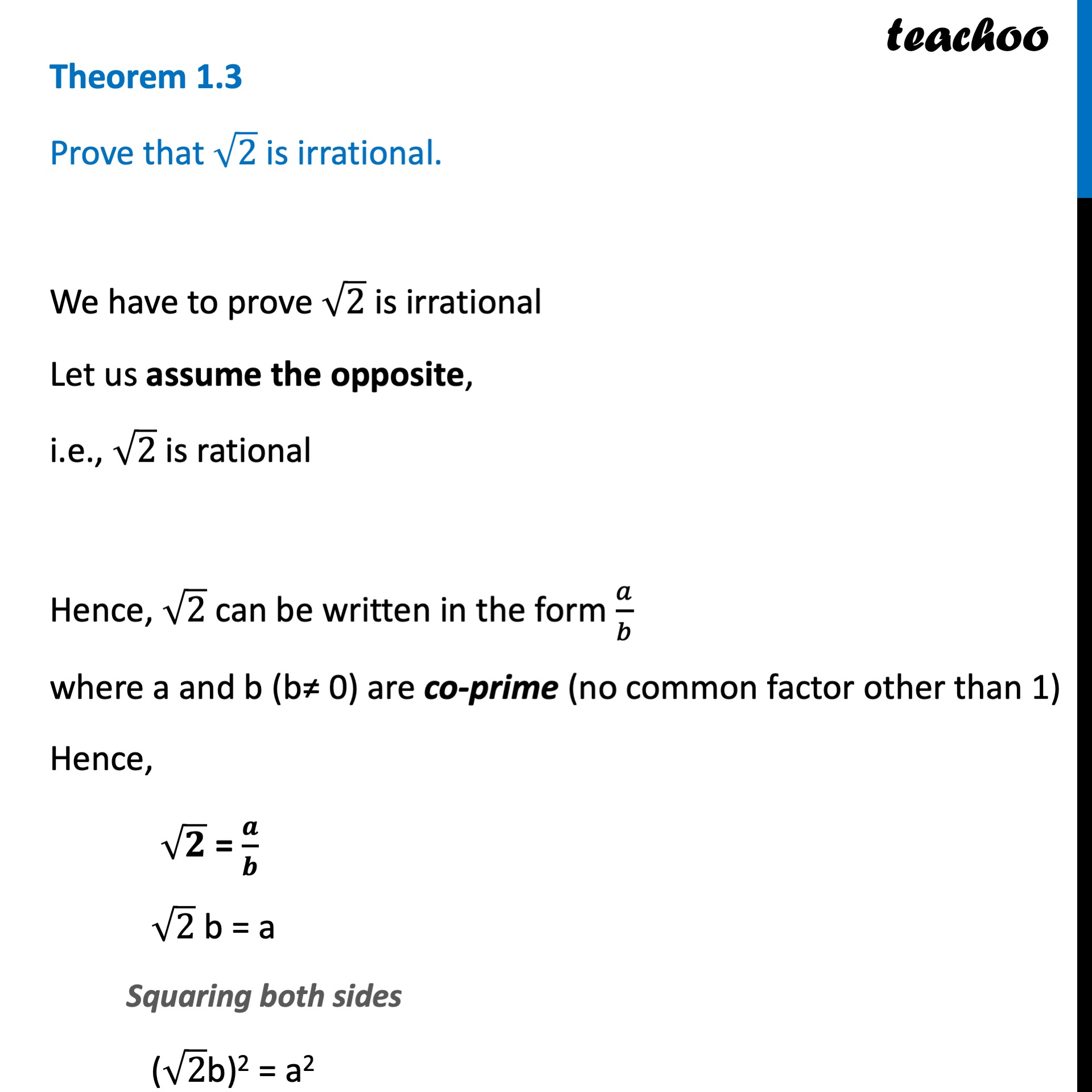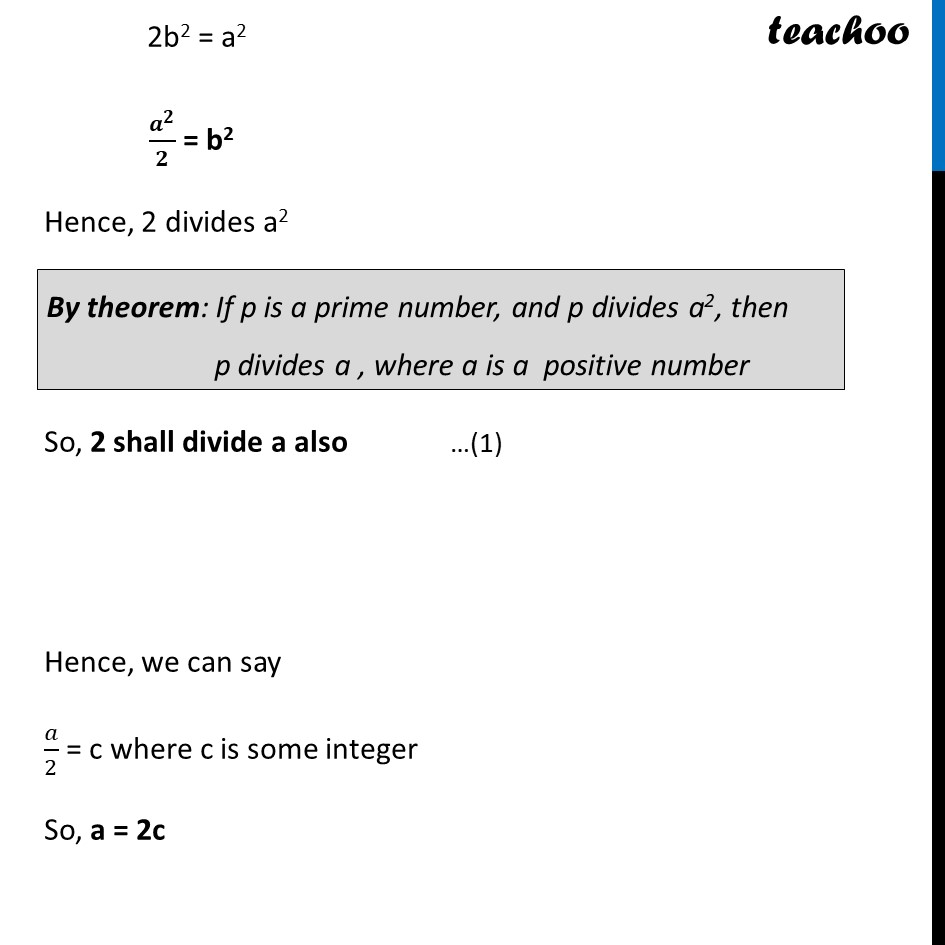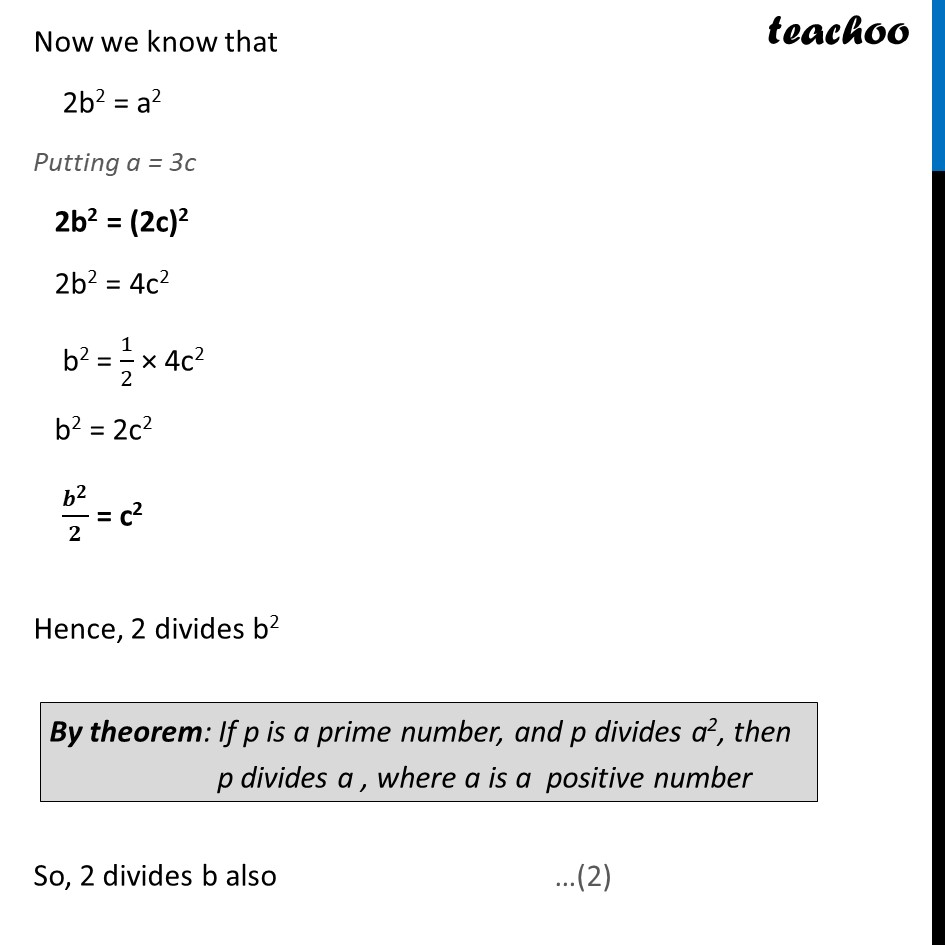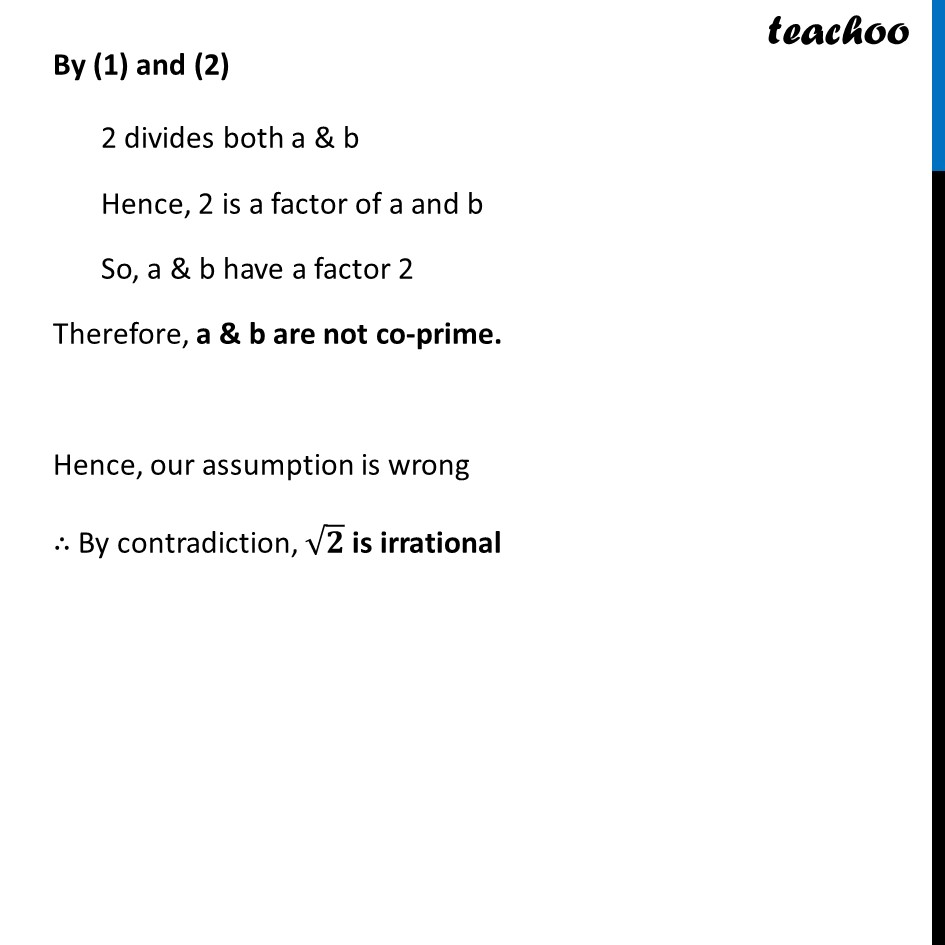Examples

Chapter 1 Class 10 Real Numbers
Serial order wiseLearn in your speed, with individual attention - Teachoo Maths 1-on-1 Class

### Transcript

Theorem 10.4 Prove that √2 is irrational. We have to prove √2 is irrational Let us assume the opposite, i.e., √2 is rational Hence, √2 can be written in the form 𝑎/𝑏 where a and b (b≠ 0) are co-prime (no common factor other than 1) Hence, √𝟐 = 𝒂/𝒃 √2 b = a Squaring both sides (√2b)2 = a2 2b2 = a2 𝒂^𝟐/𝟐 = b2 Hence, 2 divides a2 So, 2 shall divide a also Hence, we can say 𝑎/2 = c where c is some integer So, a = 2c Now we know that 2b2 = a2 Putting a = 3c 2b2 = (2c)2 2b2 = 4c2 b2 = 1/2 × 4c2 b2 = 2c2 𝒃^𝟐/𝟐 = c2 Hence, 2 divides b2 So, 2 divides b also By (1) and (2) 2 divides both a & b Hence, 2 is a factor of a and b So, a & b have a factor 2 Therefore, a & b are not co-prime. Hence, our assumption is wrong ∴ By contradiction, √𝟐 is irrational